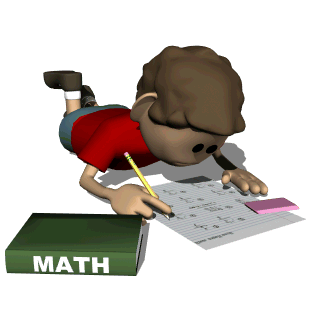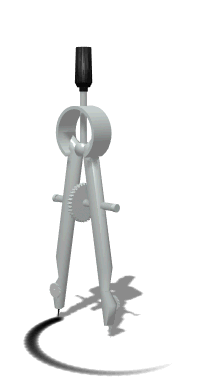• Math ReviewThe New York State Math Exam will be in the spring

Even though there are no specific things your child can study, here are some important things to remember and topics that he/she may see the day of the exam.

FACTS
Have your child practice his/her multiplication facts on a daily basis

Basic Operations
Subtract
Multiply
Divide

GEOMETRY
Measure using a protractor and a centimeter ruler
Review the different polygons
The sum of the angles of a triangle is 180 and the sum of the angles of a ploygon is 360
Perimeter is the measure around the outside
Area is the measure of the inside in square units
Similar figures are the same shape, but NOT the same size
Congruent figures are the same shape and same size
Drawing lines of symmetry

Place Value
Know the names and values from hundred thousandth to hundred million

Order of Operations
P  arentheses
E  xponents
M  ultiplication
D  ivision
A  ddition
S  ubtraction

Fractions
Simplify to the lowest term
Find a common denominator
Compare smaller and larger fractions
Convert to decimals (top goes in the box)
Change mixed numbers to improper fractions (do the circle)
Change improper fractions to mixed numbers (the top goes in the box)

Decimals
Place in order
Convert to fractions
Convert to percents (move the decimal 2 places to the right)

Percents
Convert to fractions
Convert to decimals (move the decimal 2 places to the left)

Mean
Finding an average

Line Graph
Make sure to include title, scale, labels...

..................................Steps for Writing a GOOD  answer

2.  Tell what you KNOW (I know...use formulas, definitions, explanations)

3.  Tell WHAT and WHY (So, I...use numbers and operations)

..................................

Important Vocabulary that you should know

Solution                    Solve                        Exponent
Product                    Sum                         Quotient
Difference                 Simplify                   Value
Mean                        Median                     Mode
Average                    Area                         Perimeter
Volume                     Coordinates              Diameter
Ratio                        Percent                     Of
Lowest Terms           Estimate                   LabelCheck the "Website Wonder" section for online review activities!